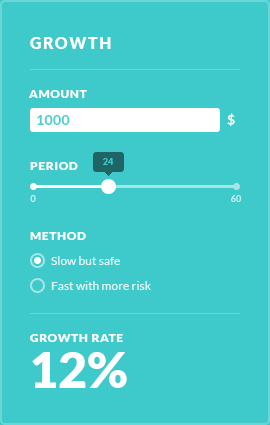# Dog year calculator

Have you ever wondered how old is your dog in human years? This dog age calculator determines the age of your dog in equivalent human years or calendar years by weight range.

### Formula

• #### Small dog age

if ((Age of dog == 1) then (Dog size + Age of dog + 10) else (Dog size + (Age of dog * 4) + 12))

• #### Medium dog age

if ((Age of dog == Medium, 21-50 lbs) then (Dog size + (Age of dog + 5) + 7) else (Small dog age)

• #### Large dog age

if ((Age of dog == Large, more then 50 lbs) then (Dog size + (Age of dog + 5) + 9) else (Small dog age)

### Works best for

This dog age calculator calculates the dog age in human years.